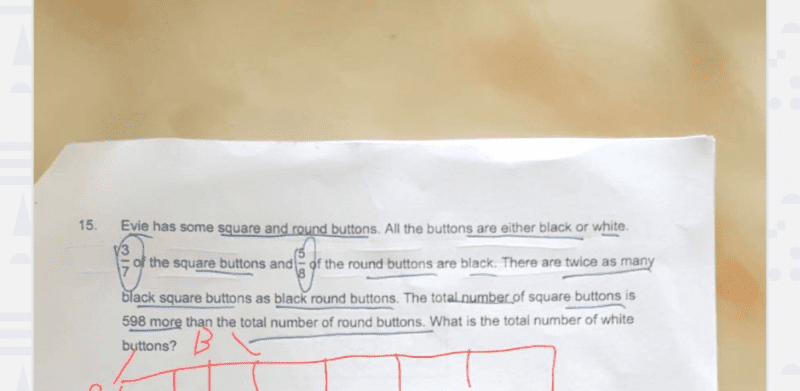# QuestionBoy, this is a tricky question.  Let me try to solve it in algebra.

Let S be the total number of Square buttons.
Let R be the total number of Round buttons.
Let B be the total number of Black buttons.
Let W be the total number of White buttons.

Firstly, we are given
(1) 3/7 S + 5/8 R = B

So this means that
(2) 4/7 S + 3/8 R = W

Now, we are given
(3) 3/7 S = 2 (5/8) R

Also, it is said that
(4) S = 598 + R

Substituting equation (4) into (3), we get
3/7 (598 + R) = (5/4) R
=> 598 + R = (7/3)(5/4)R
=> 598 + R = (35/12) R
=> 598 = (23/12) R
So, R = (12/23) 598 = 312

And S = 598 + 312 = 910

From equation (2), W = 4/7 S + 3/8 R
=> W = 4/7 (910) + 3/8 (312) = 520 + 117 = 637 #

0 Replies 1 Like